# Polynomials-NCERT Solutions

Class X Math
NCERT Solutions for Polynomials
Exercise– 2.1
Q1.  The graphs of y = p(x) are given in the figure given below. for some polynomials p (x). Find the number of zeroes of p (x), in each case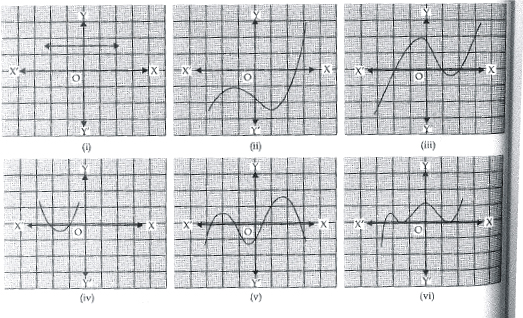Sol.  (i)  The given graph is parallel to x-axis. It does not intersect the x-axis.
•  It has no zeroes.
(ii)  The given graph intersects the x-axis at one point only.
•  It has one zero.
(iii)  The given graph intersects the x-axis at three points.
•  It has three zeroes.
(iv)  The given graph intersects the x-axis at two points.
•  It has two zeroes.
(v)  The given graph intersects the x-axis at four points.
•  It has four zeroes.
(vi)  The given graph meets the x-axis at three points.
•  It has three zeroes.
NCERT TEXTBOOK QUESTIONS SOLVED
Exercise– 2.2
Q1.  Find the zeroes of the following quadratic polynomials and verify the relationship between the zeroes and the coefficients.
(i)  x2 – 2x – 8
(ii)  4s2 – 4s + 1
(iii)  6x2 – 3 – 7x
(iv)  4u2 + 8u
(v)  t2 – 15
(vi)  3x2 – x – 4
Sol.  (i)  x2 – 2x – 8
We have p(x) = x2 – 2x – 8
= x2 + 2x – 4x – 8 = x (x + 2) – 4 (x + 2)
= (x – 4) (x + 2)
For p(x) = 0, we have
(x – 4) (x + 2) = 0
Either x – 4 = 0 ⇒ x = 4
or x + 2 = 0 ⇒ x = – 2
∴ The zeroes of x2 2x – 8 are 4 and –2.
Now, sum of the zeroes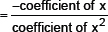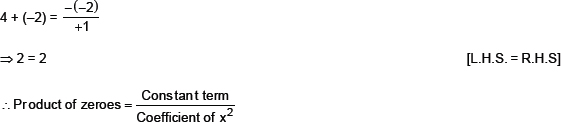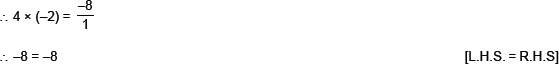Thus, relationship between zeroes and the coefficients in x2 – 2x – 8 is verified.
(ii)  4s2 – 4s + 1
We have p(s) = 4s2 – 4s + 1
= 4s2 – 2s – 2s + 1 = 2S (2S – 1) –1 (2s – 1)
= (2s – 1) (2s – 1)
For p(s) = 0, we have,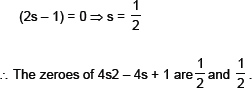Now,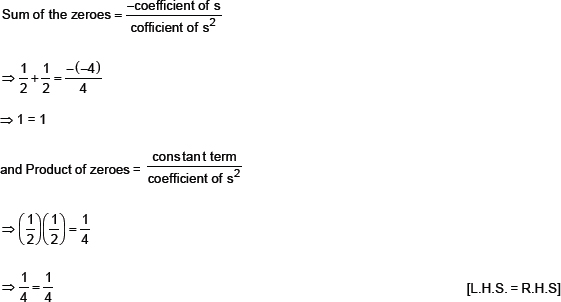Thus, the relationship between the zeroes and coefficients in the polynomial 4s2 – 4s + 1 is verified.
(iii)  6x2 – 3 – 7x
We have
p (x) = 6x2 – 3 – 7x
= 6x2 – 7x – 3
= 6x2 – 9x + 2x – 3
= 3x (2x – 3) + 1 (2x – 3)
= (3x + 1) (2x – 3)
For p (x) = 0, we have,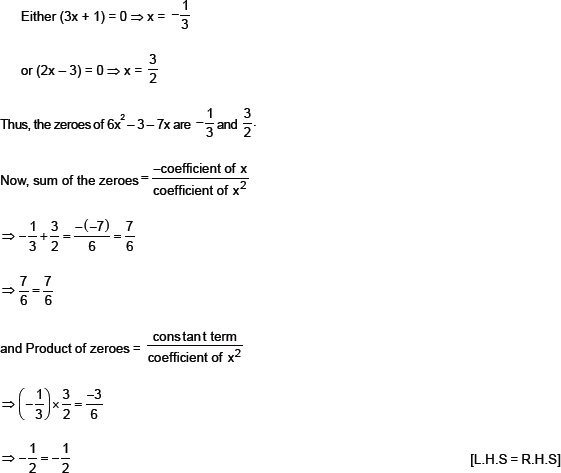Thus, the relationship between the zeroes and the coefficients in the polynomial 6x2 – 3 – 7x is verified.
(iv)  4u2 + 8u
We have, f(u) = 4u2 + 8u = 4u (u + 2)
For f(u) = 0,
Either 4u = 0 ⇒ u = 0
or u + 2 = 0 ⇒ u = –2
∴ The zeroes of 4u2 + 8u and 0 and –2
Now, 4u2 + 8u can be written as 4u2 + 8u + 0.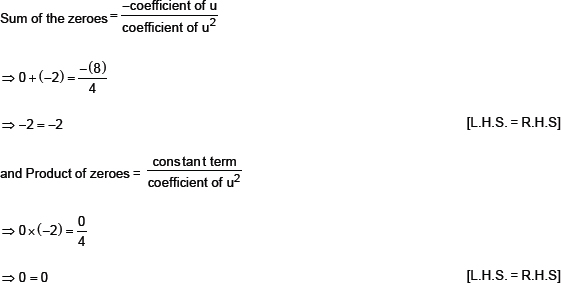Thus, the relationship between the zeroes and the coefficients in the polynomial 4u2 + 8u is verified.
(v)  t2 – 15
We have,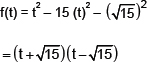For f(t) = 0, we have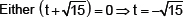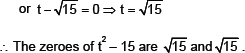Now, we can write t2 – 15 as t2 + 0t – 15.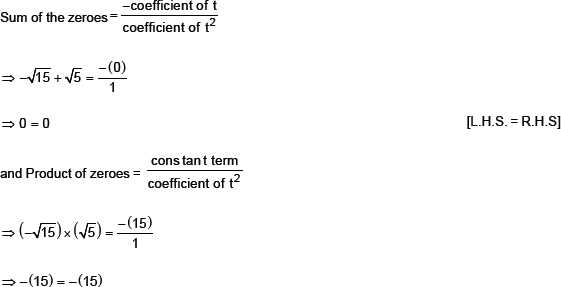Thus, the relationship between the zeroes and the coefficients in the polynomial t2 – 15 is verified.
(vi)  3x2 – x – 4
We have,
f(x) = 3x2 – x – 4 = 3x2 + 3x – 4x – 4
= 3x (x + 1) –4 (x + 1)
= (x + 1) (3x – 4)
For f(x) = 0 ⇒ (x + 1) (3x – 4) = 0
Either (x + 1) = 0 ⇒ x = – 1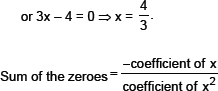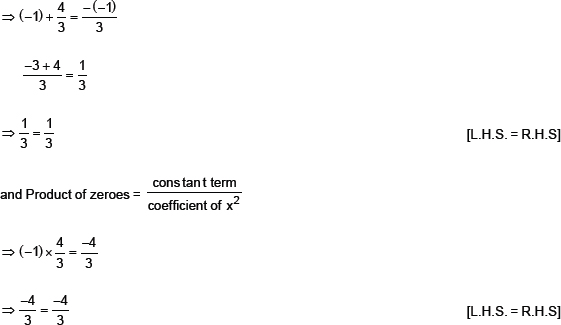Thus, the relationship between the zeroes and the coefficients in 3x2 – x – 4 is verified.
Q2.  Find a quadratic polynomial each with the given numbers as sum and product of its zeroes respectively: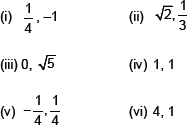Sol.
Note:
A quadratic polynomial whose zeroes are α and β is given by
p(x) = {x2 – (α + β) x + αβ}
p(x) = {x2 – (sum of the zeroes) x – (product of the zeroes)}
(i)  Since, sum of the zeroes,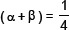Product of the zeroes, α β = –1
∴ The required quadratic polynomial is
x2 – (α + β) x + αβ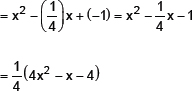Since,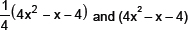have same zeroes,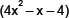is the required quadratic polynomial.
(ii)  Since, sum of the zeroes,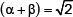Product of zeroes,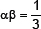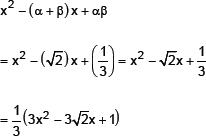Since,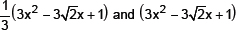have same zeroes,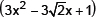is required quadratic polynomial.
(iii)  Since, sum of zeroes, (α + β) 0
Product of zeroes, αβ = 5
∴ The required quadratic polynomial is
x2 – (α + β) x + αβ
= x2 – (0) x + 5
= x2 + 5
(iv)  Since, sum of the zeroes, (α + β) = 1
Product of the zeroes = 1
∴ The required quadratic polynomial is
x2 – (α + β) x + αβ
= x2 – (1) x + 1
= x2 – x + 1
(v)  Since, sum of the zeroes,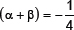Product of the zeroes∴ The required quadratic polynomial is
x2 – (α + β) x + αβ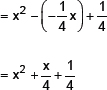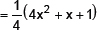Since,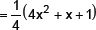and (4x2 + x + 1) have same zeroes, the required quadratic polynomial is (4x2 + x + 1).
(vi)  Since, sum of the zeroes, (α + β) = 4
Product of the zeroes, αβ = 1
∴ The required quadratic polynomial is
x2 – (α + β) x + αβ
= x2 – (4)x + 1
= x2 – 4x + 1
Exercise– 2.3
Q1.  Q1. Divide the polynomial p (x) by the polynomial g(x) and find the quotient and remainder in each of the following:
(i)  p (x) = x3 – 3x2 + 5x – 3, g (x) = x2 – 2
(ii)  p (x) = x4 – 3x2 + 4x + 5, g (x) = x2 + 1 – x
(iii)  p (x) = x3 – 5x + 6, g (x) = 2 – x2
Sol.  Here, dividend p (x) = x3 – 3x2 + 5x – 3
divisor g(x) = x2 – 2
∴ We have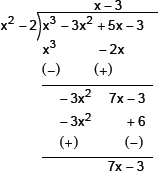Thus, the quotient = (x – 3) and remainder = (7x – 9)
(iii)  Here, dividend p(x) = x4 – 3x2 + 4x + 5
and divisor g(x) = x2 + 1 – x
= x2 – x + 1
∴ We have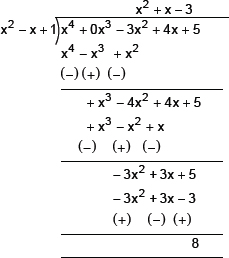Thus, the quotient is (x2 + x – 3) and remainder = 8
(iii)  Here, divided, p(x) = x4 – 5x + 6
and divisor, g(x) = 2 – x2
∴ We have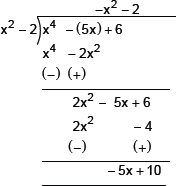Thus, the quotient = –x2 – 2 and remainder = –5x + 10.
Q2.  Check whether the first polynomial is a factor of the second polynomial by dividing the second polynomial by the first polynomial:
(i)  t2 – 3;                 2t4 + 3t3 – 2t2 – 9t – 12
(ii)  x2 + 3x + 1;                                  3x4 + 5x3 – 7x2 + 2x + 2
(iii)  x3 + 3x + 1;                                  x5 + 4x3 + x + 3x + 1
Sol.  (i)  Dividing 2t4 + 3t3 – 2t2 – 9t – 12 by t2 – 3,
We have: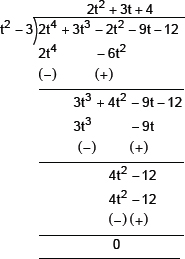∴ Remainder = 0
∴ (t2 – 3) is a factor of 2t4 + 3t3 – 2t2 – 9t – 12.
(ii)  Dividing 3x4 + 5x3 – 7x2 + 2x + 2 by
x2 + 3x + 1, we have: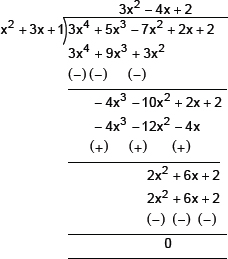∴ Remainder = 0.
∴ x2 + 3x + 1 is a factor of 3x4 + 5x3 – 7x2 + 2x + 2.
(iii)  Dividiing x5 + 4x3 + x + 3x + 1, we get: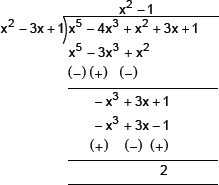∴ The remainder = 2, i.e., remainder ≠ 0
∴ x3 – 3x + 1 is not a factor of
x5 – 4x3 + x2 + 3x + 1.
Q3.  Obtain all other zeroes of 3x4 + 6x3 – 2x2 – 10x – 5, if two of its zeroes are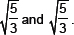Sol.  We have p(x) = 3x4 + 6x3 – 2x2 – 10x – 5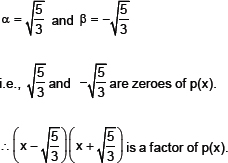Now, let us divide 3x4 + 6x3 – 2x2 – 10x – 5 by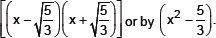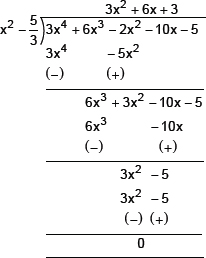∴ 3x4 + 6x3 – 2x2 – 10x – 5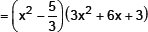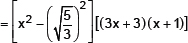For p(x) = 0, we have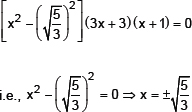or 3x + 3 = 0 ⇒ x = –1
or x + 1 = 0 ⇒ x = –1
Thus, all the other zeroes of the given polynomial are –1 and –1.
Q4.  On dividing x3 – 3x2 + x + 2 by a polynomial g(x), the quotient and remainder were x – 2 and –2x + 4 respectively. Find g(x).
Sol.  Here,
Dividened p(x) = x3 – 3x2 + x + 2
Divisor = g(x)
Quotient = (x – 2)
Remainder = (–2x + 4)
Since,
(Quotient – Divisor) + Remainder = Dividend
∴ [(x – 2)] – g(x)] + [(–2x + 4)] = x3 – 3x2 + x + 2
⇒ (x – 2) – g(x) = x3 – 3x2 + x + 2 – (–2x + 4)
= x3 – 3x2 + x + 2 + 2x – 4
= x3 – 3x2 + 3x – 2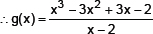Now, dividing x3 – 3x2 + 3x – 2 by x – 2,
We have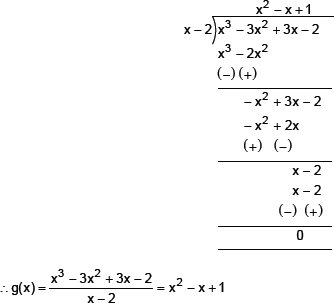Thus, the required divisor g(x) = x2 – x + 1.
Q5.  Give example of polynomials p(x), g(x), q(x) and r(x), which satisfy the division algorithm and
(i)  deg p(x) = deg q (x)
(ii)  deg q(x) = deg r (x)
(iii)  deg r (x) = 0
Sol. We can have
(i)  p(x) = 3x2 – 6x + 27
g(x) = 3
q(x) = x2 – 2x + 9
r(x) = 0
⇒ p(x) = q(x) × g(x) + r(x).
(ii)  p(x) = 2x3 – 2x2 + 2x + 3
g(x) = 2x2 – 1
q(x) = x – 1
r(x) = 3x + 2
⇒ p(x) = q(x) × g(x) + r(x)
(iii)  p(x) = 2x3 – 4x2 + x + 4
g(x) = 2x2 + 1
q(x) = x – 2
r(x) = 6
⇒ p(x) = q(x) × g(x) + r(x)
Exercise– 2.4
Q1.  Verify that the numbers given alongside of the cubic polynomials below are their zeroes. Also verify the relationship between the zeroes and the coefficients in each case:
(i)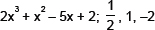(i)  x3 – 4x2 + 5x – 2; 2, 1, 1
Sol.  (i)  ∵ p(x) = 2x3 + x2 – 5x + 2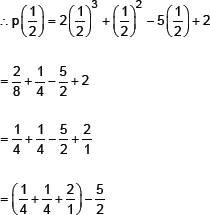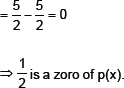Again,
p(1) = 2(1)3 + (1)2 – 5(1) + 2
= 2 + 1 – 5 + 2
= (2 + 2 + 1) – 5
= 5 – 5 = 0
⇒ 1 is a zero of p(x).
Also,
p(–2) = 2(–2)3 + (–2)2 –5 (–2) + 2
= 2(–8) + (4) + 10 + 2
= –16 + 4 + 10 + 2
= –16 + 16 = 0
⇒ –2 is a zero of p(x).
Relationship
∵p(x) = 2x3 + x2 – 5x + 2
∴Comparing it with ax3 + bx2 + cx + d, we have:
a = 2, b = 1, c = – 5 and d = 2
Also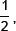1 and – 2 are the zeroes of p(x)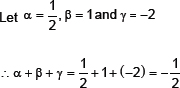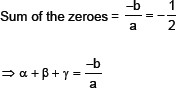Sum of product of zeroes taken in pair: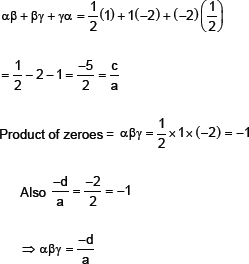Thus, the relationship between the co-efficients and the zeroes of p(x) is verified.
(ii)  Here, p(x) = x3 – 4x2 + 5x – 2
p(2) = (2)3 – 4(2)2 + 5(2) – 2
= 8 – 16 + 10 – 2
= 18 – 18 = 0
⇒ 2 is a zero of p(x).
Again p(1) = (1)3 – 4(1)2 + 5(1) – 2
= 1 – 4 + 5 – 2
= 6 – 6 = 0
⇒ 1 is a zero of p(x).
∴2, 1, 1 are zeroes of p(x).
Now, comparing
p(x) = x3 – 4x2 + 5x – 2 with ax3 + bx2 +cx + d = 0, we have
a = 1, b = –4, c = 5 and d = –2
∵2, 1, 1 are the zeroes of p(x)
Let
α = 2
β = 1
γ = 1
Relationship, α + β + γ = 2 + 1+ 1 = 4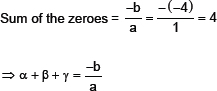Sum of product of zeroes taken in pair:
αβ + βγ + γα = 2(1) + 1(1) + 1(2)
= 2 + 1 + 2 = 5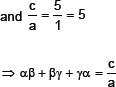⇒ αβ + βγ + γα =
Product of zeroes = αβγ = (2) (1) (1) = 2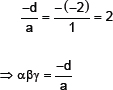Thus, the relationship between the zeroes and the co-efficients of p(x) is verified.
Q2.  Find the cubic polynomial with the sum, of the products of its zeroes taken two at a time and the product of its zeroes as 2, –7, –14 respectively.
Sol.  Let the required cubic polynomial be ax3 + bx2 + cx + d and its zeroes be α, β and γSince,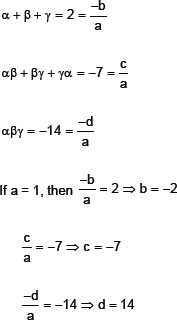∴The required cubic polynomial
= 1x3 + (–2)x2 + (–7)x + 14
= x3 – 2x2 – 7x + 14
Q3.  If the zeroes of the polynomial x3 – 3x2 + x + 1 are a – b, a and a + b then find ‘a’ and ‘b’.
Sol.  We have
p(x) = x3 – 3x2 + x + 1
comparing it with Ax3 + Bx2 + Cx + D.
We have
A = 1, B = –3, C = 1 and D = 1
∵It is given that (a – b), a and (a + b) are the zeroes of the polynomial.
∴Let,
α = (a – b)
β = a
and γ = (a + b)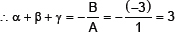⇒ (a – b) + a + (a + b) = 3
⇒ 3a = 3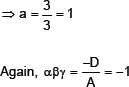⇒ (a – b) × a × (a + b) = –1
⇒ (1– b) ×1× (1 + b) = –1
⇒ 1– b2 = –1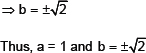Q4.  If two zeroes of the polynomial x4 6x3 – 26x2 + 138x – 35 are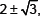find other zeroes.
Sol.  Here, p(x) = x4 – 6x3 – 26x2 + 138x – 35.
∴Two of the zeroes of p (x) are: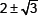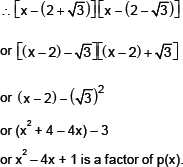Now, dividing p (x) by x2 – 4x + 1, we have: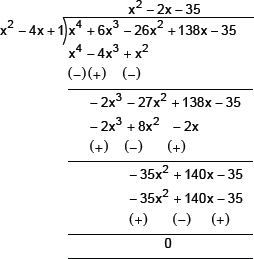∴(x2 – 4x + 1)(x2 – 2x – 35) = p(x)
⇒ (x2 – 4x + 1) (x – 7) (x + 5) = p(x)
i.e., (x – 7) and (x + 5) are other factors of p(x).
∴7 and –5 are other zeroes of the given polynomial.
Q5.  If the polynomial x4 – 6x3 + 16x2 – 25x + 10 is divided by another polynomial x2 – 2x + k, the remainder comes out to be (x + α ), find k and α.
Sol.  Applying the division algorithm to the polynomials x4 – 6x3 + 16x2 – 25x + 10 and x2 – 2x + k, we have: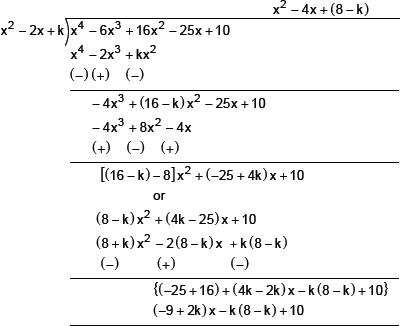∴Remainder = (2k – 9) x – k(8 – k) + 10
But the remainder = x + α
Therefore, coparing them, we have: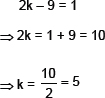and
α = –k(8 – k) + 10
= –5(8 – 5) + 10
= –5(3) + 10
= –15 + 10
= –5
Thus, k = 5 and α = –5